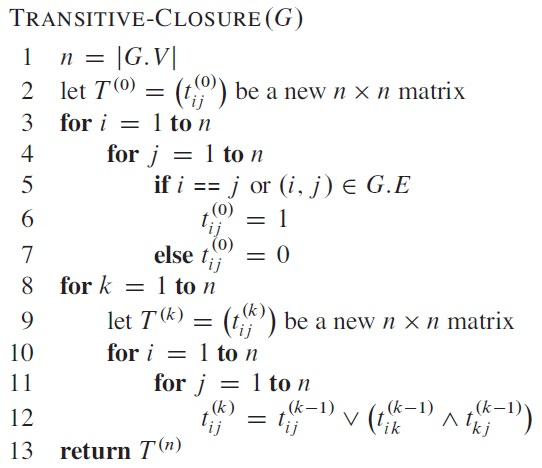CIS 5511. Programming Techniques

Graphs (2)

### 1. Single-source shortest paths

In a weighted graph, one type of optimization problem is to find the shortest path between vertices (one-to-one, one-to-many, many-to-many). Here "distance" or "weight" can represent many different things, so can be any finite (positive, negative, or zero) value.

The weight of a path is the sum of the weights of the edges in the path. A shortest path is a path whose weight has the lowest value among all paths with the same source and destination vertex. A section of a shortest path is also a shortest path.

In general, a shortest path can be defined in graphs that are either directed or undirected, with or without cycle. However, a shortest path cannot contain cycle. Therefore, for graph G = (V, E), the shortest path contains less than |V| edges. Example: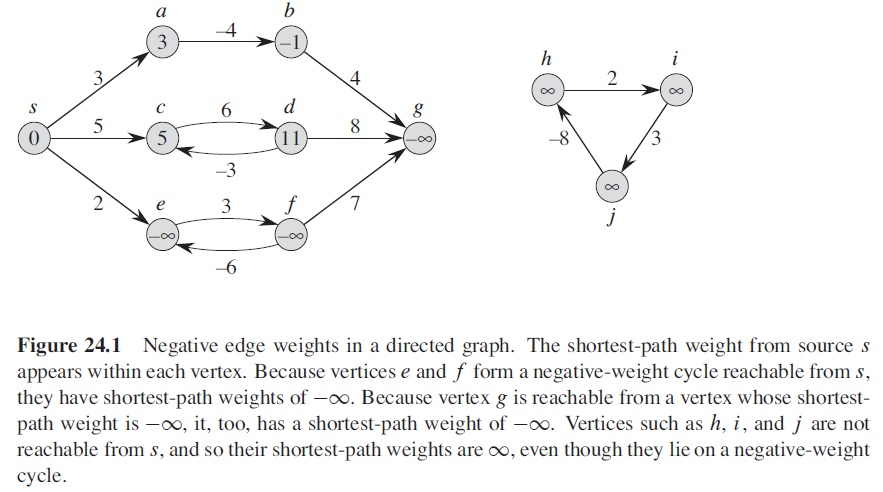A trivial solution to the problem is to enumerate all possible paths (if there are finite), and compare their distances. However, this solution is usually too time-consuming to be useful.

If all edges are equally-weighted, the breadth-first search algorithm introduced previous finds shortest paths from a source vertex to all other vertices. However, it does not work if the edges have different weights.

To represent the shortest paths, we use an array d for the shortest distance, found so far, between each vertex and the source, and an array π for the predecessor of each vertex on the path. The following algorithm initialize the data structure.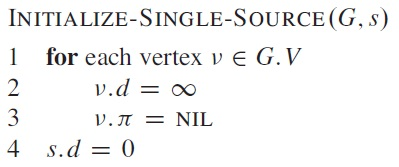Many shortest-path algorithm use the "relaxation" technique, which maintains the shortest paths to v found so far, and try to improve them when a new vertex u is taken into consideration. The matrix w stores the weights of the edges.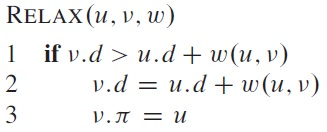There are various ways to use relax to get the shortest paths.

Bellman-Ford algorithm processes the graph |V| − 1 passes, and in each pass, tries the edges one-by-one to relax the distance. After that, if there is still possible relaxation, the graph contains negatively-weighted cycle.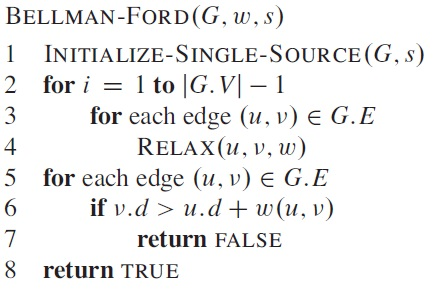The running time is O(V E).

Example: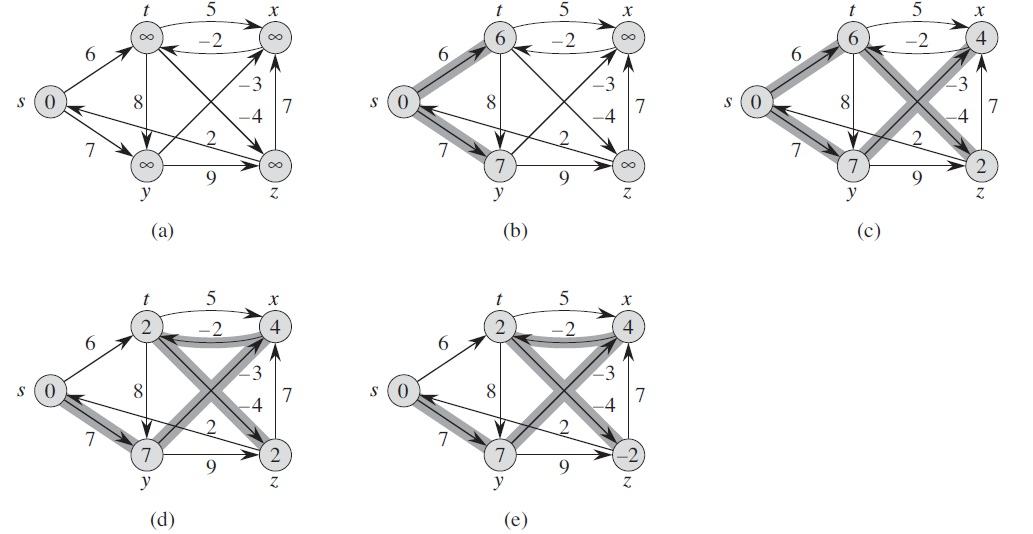If the graph is a DAG, there are faster solutions. The following algorithm topologically sort the vertices first, then determine the shortest paths for each vertex in that order. It runs in Θ(V + E) time, which comes from topological sorting (and DFS).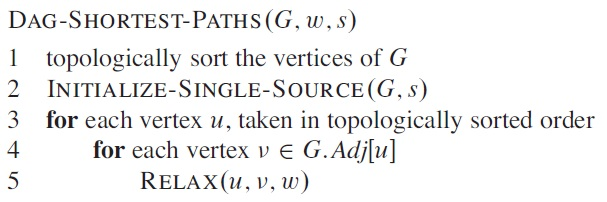Example: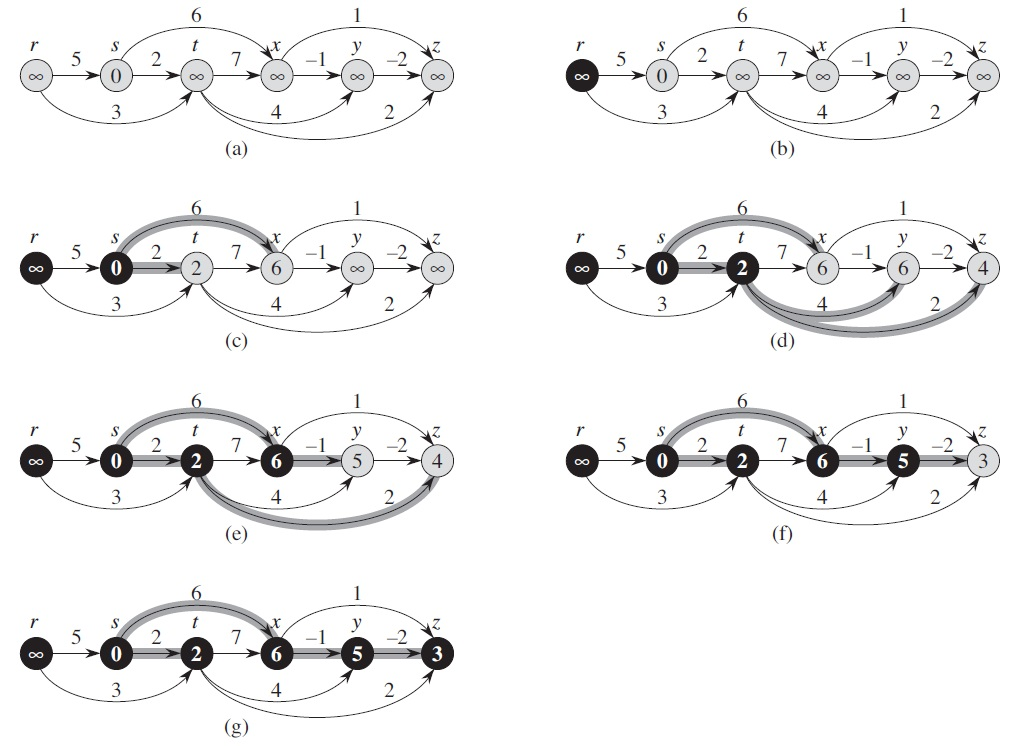Dijkstra's algorithm works for directed graphs without negative weight. It repeatedly selects the vertex with the shorted path, then use it to relax the paths to other vertices.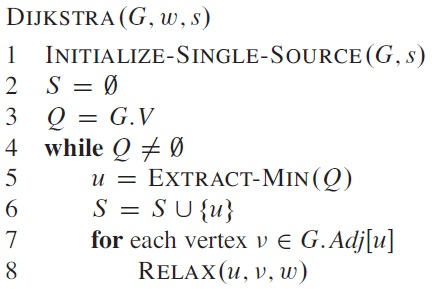This is a greedy algorithm. Its running time is O((V + E) lg V).

Example: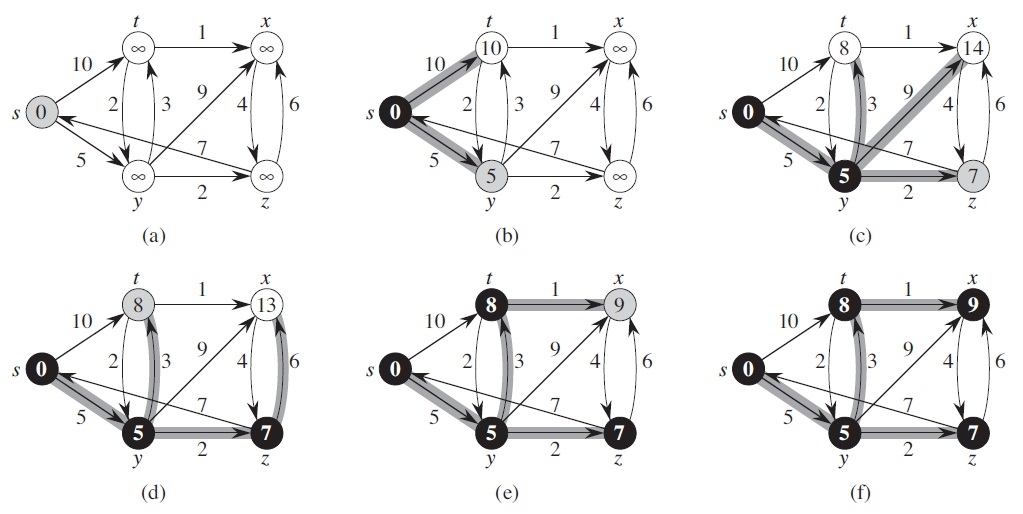### 2. All-pairs shortest paths

If we want the shortest paths between every pair of vertices, it is inefficient to repeat an algorithm for single-source.

Many algorithms use the adjacency matrix to remember the shortest paths found so far, and a predecessor matrix Π, where πij is the predecessor of j on some shortest path from i to j. Given it, the following algorithm prints the shortest path.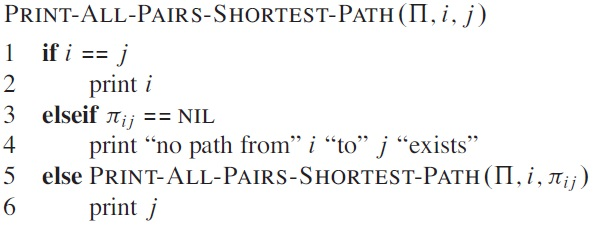The following dynamic-programming algorithm extends shortest paths starting with single edges, and in each step tries to add one more edge.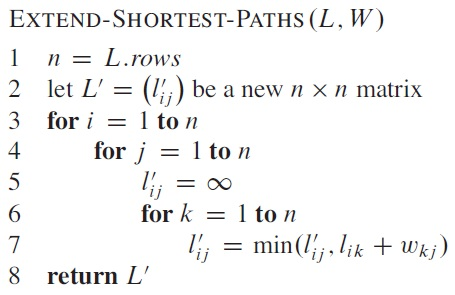Each call to the above algorithm will extend the length of path under consideration by one. Therefore, if we start with L = W, and repeatedly call the algorithm n−1 times, we will get a matrix for the shortest paths. This solution takes Θ(n4) time. Algorithm: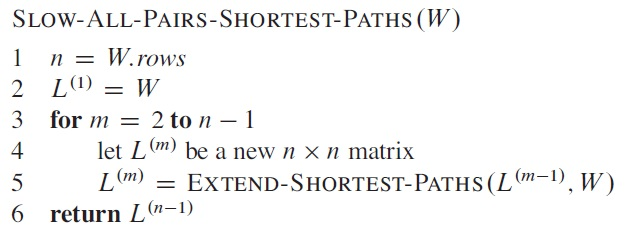Example: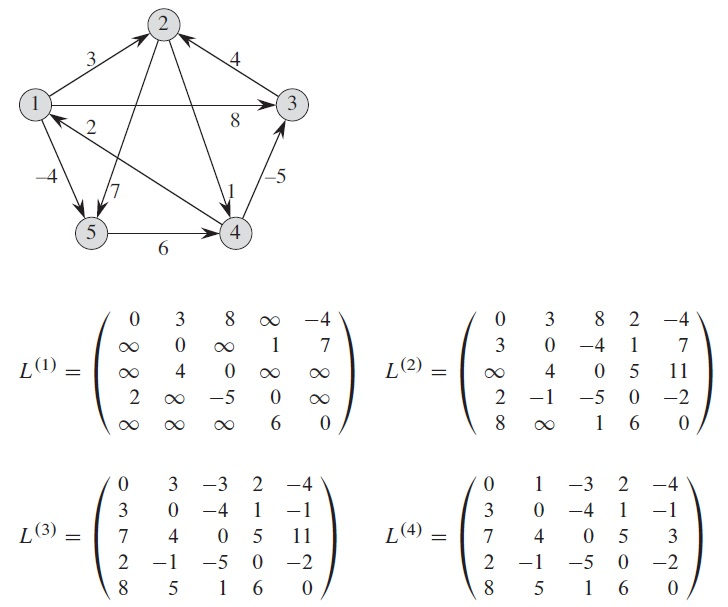The above algorithm can be improved by updating the loop variable not as m = m+1 but as m = 2m, and change the W in line 5 to L(m−1), so as to achieve Θ(n3 lg n) time.

Floyd-Warshall algorithm in each step adds one possible intermediate vertex into the shortest paths.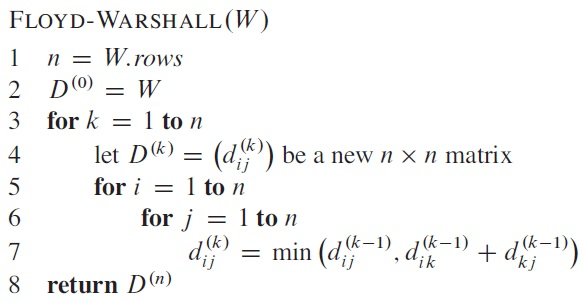For the same problem, the intermediate results are: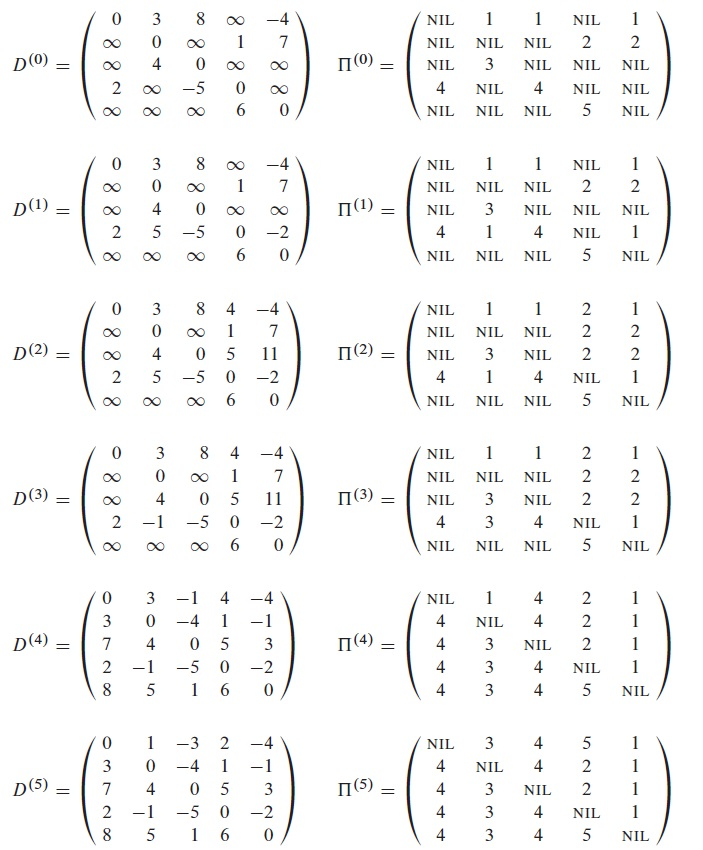The running time of the above algorithm is Θ(n3).

A similar algorithm calculates the transitive closure of a graph, where T(n)ij = 1 if and only if there is a path from i to j.